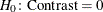#### Analysis of Contrasts

If you specify a CONTRAST statement, PROC SURVEYREG produces an Analysis of Contrasts table, which displays Wald’s F test for the contrast. If you use more than one CONTRAST statement, the procedure displays all results in the same table. The Analysis of Contrasts table contains the following information for each contrast:

• Contrast, which is the label of the contrast

• Num DF, which is the numerator degrees of freedom for Wald’s F test

• F Value, which is Wald’s F statistic for testing• Pr > F, which is the significance probability corresponding to the F Value